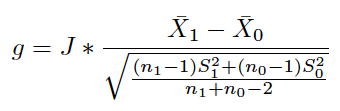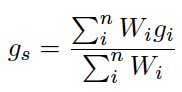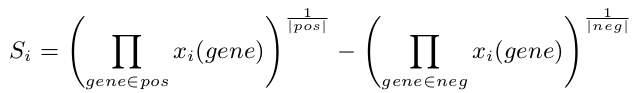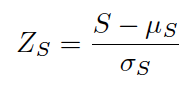# Contents

## 0.1 Introduction

The MetaIntegrator package comprises several analysis and plot functions to perform integrated multi-cohort analysis of gene expression data (meta-analysis). The advent of the gene expression microarray has allowed for a rapid increase in gene expression studies. Largely due to the MIAME standards for data sharing, many of these studies have been deposited into public repositories such as the NIH Gene Expression Omnibus (GEO) and ArrayExpress. There is now a wealth of publicly available gene expression data available for re-analysis.

An obvious next step to increase statistical power in detecting changes in gene expression associated with some condition is to aggregate data from multiple studies. However, inter-study technical and biological differences prevent us from simply pooling results and summarizing our findings. A random-effects model of meta-analysis circumvents these issues by assuming that the results from each study is drawn from a single distribution, and that such inter-study differences are thus a ‘random effect’. Thus, the MetaIntegrator package will perform a DerSimonian & Laird random-effects meta-analysis for each gene (not probeset) between all target studies between cases and controls; it also performs a Fischers sum-of-logs method on the same data, and requires that a gene is significant by both methods. The resulting p-values are False discovery rate (FDR) corrected to q-values, and will evaluate the hypothesis of whether each gene is differentially expressed between cases and controls across all studies included in the analysis.

The resulting list of genes with significantly different expression between cases and controls can be used for multiple purposes, such as (1) a new diagnostic or prognostic test for the disease of interest, (2) a better understanding of the underlying biology, (3) identification of therapeutic targets, and multiple other applications. Our lab has already used these methods in a wide variety of diseases, including organ transplant reject, lung cancer, neurodegenerative disease, and sepsis (Khatri et al., J Exp Med 2013; Chen et al, Cancer Res 2014; Li et al., Acta Neur Comm 2014; Sweeney et al, Sci Trans Med 2015).

The MetaIntegrator Vignette will take the user through the basic steps of the package, including basic multi-cohort analysis, leave-one-out (LOO) analysis (whereby each of the included datasets is left out and multi-cohort analysis is run on the remaining datasets in a round-robin fashion), selection of significant genes, and then analysis of the gene set. The MetaIntegrator package assumes that the user (1) already has their data in hand, and (2) has already decided which datasets to include in the multi-cohort meta-analysis. Our group recommends that some datasets be left out of the analysis, if possible, for independent validation.

Contact
Winston A. Haynes

Links

Installation

``install.packages("MetaIntegrator")``

## 0.2 The Meta-Analysis Algorithm

### 0.2.1 Meta-analysis of gene expression data

The Metaintegrator package can be used to run a meta-analysis on microarray gene expression data as described in Khatri et al. J Exp Med. 2013. Briefly, it computes an Hedges’ g effect size for each gene in each dataset defined as:Eq1

where \(1\) and \(0\) represent the group of cases and controls for a given condition, respectively. For each gene, the summary effect size \(g_s\) is computed using a random effect model as:Eq2

where \(W_i\) is a weight equal to \(1/(V_i+T^2)\), where \(V_i\) is the variance of that gene within a given dataset \(i\), and \(T^{2}\) is the inter-dataset variation (for details see: Borenstein M et al Introduction to Meta-analysis, Wiley 2009). For each gene, the False discovery rate (FDR) is computed and a final set of genes is selected based on FDR thresholding.

### 0.2.2 Computation of a signature score

For a set of signature genes, a signature score can be computed as:Eq3

where \(pos\) and \(neg\) are the sets of positive and negative genes, respectively, and \(x_i(gene)\) is the expression of any particular gene in sample \(i\) (a positive score indicates an association with cases and a negative score with controls). This score \(S\) is then converted into a z-score \(Z_s\) as:Eq4

## 0.3 Overview Meta-Analysis workflow

1. Data collection, curation and annotation, select datasets for discovery and validation: Helper Functions
2. Meta-analysis on discovery datasets: Meta-Analysis, Filtering, Validation, Visualization, Search, Helper Functions
3. Validation on independent validation datasets: Visualization, Validation, Helper Functions